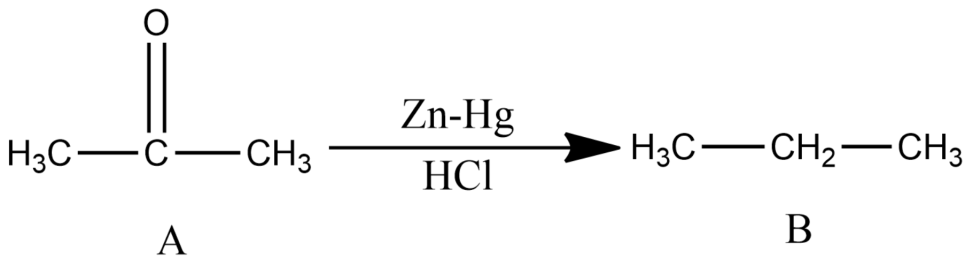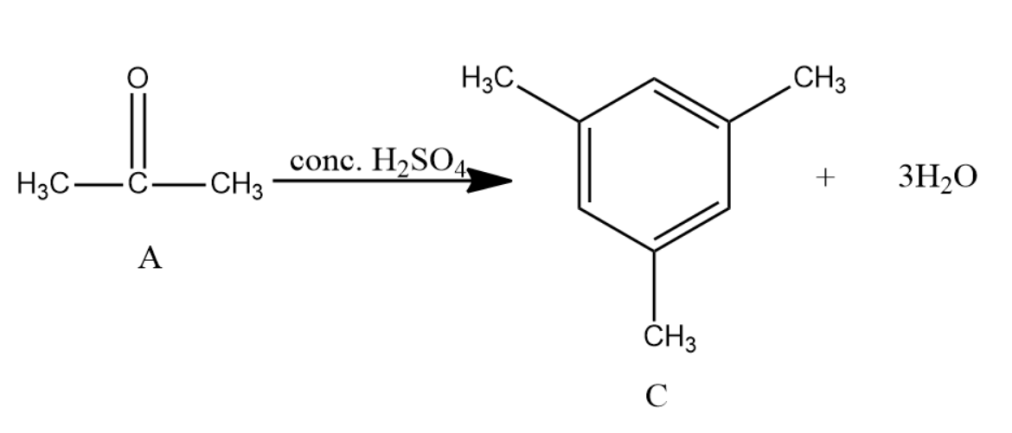QuestionAnswers

# Compound A of molecular formula ${{C}_{3}}{{H}_{6}}O$ does not reduce Tollens reagent and Fehling’s solution. Compound A undergoes Clemmensen reduction to give compound B of the molecular formula ${{C}_{3}}{{H}_{8}}$ . Compound A in the presence of conc. ${{H}_{2}}S{{O}_{4}}$ condenses giving aromatic compound C of the molecular formula ${{C}_{9}}{{H}_{12}}$ . Identify B and C. Explain the reactions.

-Since the compound, A with molecular formula ${{C}_{3}}{{H}_{6}}O$ is not reduced by Tollen’s reagent and Fehling’s solution and is undergoing Clemmensen reduction, so it must be ketone (acetone).
-Acetone is further reacted with conc. ${{H}_{2}}S{{O}_{4}}$ with and distilled water gives Mesitylene which is the compound C. It has the molecular formula as ${{C}_{9}}{{H}_{12}}$ and is an aromatic compound.